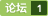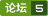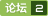在delphi里如何进行一些数据运算 如开根,平方等 [问题点数：0分]public class one { /** * @param args */ public static void main(String[] args) { // TODO Auto-generated method stub A num ; num=new A(); num.n =6; num.m =3; n
java中的开方Math.sqrt(n)函数和平方{a的b次方Math.pow(a, b)}
1，**重点内容**sqrt是开方： 比如math.sqrt(9)结果为3 意思是求9的开方根，数学上称为根号2下的9 2.**重点内容**pow（a,b） Math.pow(27, 3)意思是求27的三次方 就是27*27*27的意思 重点内容假如你想求开三次方，或者四次方 可以借助pow方法 例如：Math.pow(27, 1/3) 结果为3 就是对27的1/3

Delphi-数学运算函数与过程
Delphi的常用数学函数
Oracle SQL 之 数学计算-开方根(咋个办呢 zgbn)
Oracle SQL 之 数学计算-开方根1、求100的5次开方。
mysql计算开N次方以及求N次平方根函数
mysql<em>如何</em>计算一个数的N次方？ 函数为POWER(VALUE,N) 以开2次方为例 /**mysql 开方计算函数**/ SELECT VALUE,POWER(VALUE,2) FROM test;    求N次方根，POWER(VALUE,1/N)以2为例 /**mysql 开<em>平方</em>根函数**/ SELECT VALUE,POWER(VALUE,1/2) FROM test...
python 计算开平方
import time import math def square_(x,epsilon): assert x>=0 assert epsilon>0 low = 0 high =max(x,1.0) quess = (low+high)/2.0 ctr=1 while abs(quess**2-x)>epsilon and ctr<=1

【Java BigInteger开方sqrt的实现】

java求平方
//3的<em>平方</em> Math.pow(3, 2); //3的立方 Math.pow(3, 3);
Java BigInteger求任意n(n∈N*)次方根的实现

【关于c/c++中开平方函数sqrt()的用法】

java实现开根号的运算

python编写简单计算器，计算一个数的平方
#编写简单计算器，计算一个数的<em>平方</em>，附效果图！！！while True: a=float(input('请输入实数：')) def power(x): return x*x print(a,'^2=',power(a)) b=int(input('是否要继续计算，是，请输入1，否,请输入0:\n')) if b==0: print('已退出计算器') break else:
CSharp 常用函数
Int64.Parse 方法将数字的字符串表示形式转换为它的等效 64 位有符号整数。string s=Console.ReadLine();long num=Int64.Parse(s)

C#开根号函数：math.pow()函数

java 带根号的开平方

java中不用Math.sqrt,实现开平方根的算法

#include using namespace std; #include float Q_rsqrt( float number ) {   long i;   float x2, y;   const float threehalfs = 1.5F;     x2 = number * 0.5F;   y  = number;   i  = * ( long * )

Go语言实现大数开方程序
Go语言的big包实现大数<em>运算</em>，但是有关大整数<em>运算</em>，似乎没有相应的开方程序。 这里给出的程序，实现了大整数的开方<em>运算</em>函数。该程序是基于大整数开方<em>运算</em>的算法实现的。 Go语言程序： // bigintsqrt project main.go package main import ( "fmt" "math" "math/big" ) func main() { v :=
GO 语言用牛顿法开平方根的值
package mainimport ( &quot;fmt&quot; &quot;math&quot;)func Sqrt(x float64) float64 { z := 1.0 for { tmp := z - (z*z-x)/(2*z) if tmp == z || math.Abs(tmp-z) &amp;lt; 0.000000000001 { break } z = tmp } return z}func main...

java大数开根号问题
import java.util.*; import java.math.*; public class Main{ static BigInteger cal(BigInteger x){ BigInteger l = BigInteger.ONE ; BigInteger r = x ; BigInteger temp = BigInte

#include&amp;amp;amp;lt;stdio.h&amp;amp;amp;gt; #include&amp;amp;amp;lt;math.h&amp;amp;amp;gt; double f(double n,double x); int main() { double x,sum,n,i; scanf(&amp;amp;quot;%lf%lf&amp;amp;quot;,&amp;amp;amp;amp;x,&amp;amp;amp;amp;n); sum=f(n,x);

Python开根号
1，开<em>平方</em> Python数字sqrt()函数返回x的<em>平方</em>根(x > 0)。 语法 以下是sqrt()方法的语法 - import math math.sqrt( x ) Python 注意 - 此函数不可直接访问，需要导入math模块，然后需要使用math静态对象调用此函数。 2，开n次方 利用pow(a, b)函数即可。需要开a的r次方则pow(a, 1.0/r)。

Lua 基础之运算

(数字图像处理)腐蚀/膨胀/开运算/闭运算
(数字图像处理)腐蚀/膨胀/开<em>运算</em>/闭<em>运算</em> 腐蚀：是一种消除边界点，使边界向内部收缩的过程。可以用来消除小且无意义的物体。 膨胀：是将与物体接触的所有背景点合并到该物体中，使边界向外部扩张的过程。可以用来填补物体中的空洞。 开<em>运算</em>：先腐蚀后膨胀的过程开<em>运算</em>。用来消除小物体、在纤细点处分离物体、平滑较大物体的边界的同时并不明显改变其面积。开<em>运算</em>通常是在需要去除小颗粒噪声，以及断开目标物

Java实现开根号
Java实现开根号 二分法 static float sqr(float x){ float max = x; float min = 0; float mid=(min+max)/2; while (true){ if (Math.abs(x-mid*mid)&amp;amp;lt;0.002) return mid;...
R语言中的数学计算（转载）
R语言中的数学计算关于作者：张丹(Conan), 程序员Java,R,PHP,Javascript weibo：@Conan_Z blog: http://blog.fens.me email: bsspirit@gmail.com 转载请注明出处： http://blog.fens.me/r-mathematics/r-math前言R是作为统计语言，生来就对数学有良好的支持，一个函数就能
STM32F407IG开启FPU，做开方运算
STM32F4xx自带DSP处理器，FPU单元，做开方效率很高。但是有些设置要注意： 如图： 首先，Use FPU要选上； 然后，这些要包含进去： 检查一下你的项目里有没有这个文件，如果有，如果编译通过，恭喜你，可以用开方指令了。如图： 做了个勾股定理<em>运算</em>，求斜边。。。

<em>数据</em>PROG_X<em>平方</em><em>运算</em> 无<em>数据</em>校验，只能对整数<em>数据</em><em>进行</em>操作<em>运算</em> QQ：405418144 E_mail:sgzhou12345@sina.com

LINUX-SHELL之数字运算

14. 对一幅二值图像进行膨胀、腐蚀、开运算和闭运算，观察效果。
#include #include #include #include int main(void) { int i = 40; IplImage *img = cvLoadImage("6013202130.bmp", 0); IplImage *imga = cvLoadImage("xibao.jpg", 0); IplImag
C语言之linux内核实现平方根计算算法

numpy简单教程-数组运算与常用函数

hdu 4027(线段树区间开方求和)

MagicNumber求平方根倒数

IOS开发之----常用函数和常数

java实现开根号
java开根号
SPOJ GSS4 (区间开根号 + 区间查询) (线段树)

#include #include #include #include #include #include "util.h" #define eps 0.00001 float SqrtByBisection(float n) { if(n<0) { return -1.0; } else { float low,up,mid,last; low=0,up
Java入门学习-使用Math，实现lg、平方、开方、round、floor、ceil的算法。

&amp;lt;p&amp;gt;最近在做一个word的行业上的地区<em>数据</em>统计报告，以前的做法是把excel表里的<em>数据</em>一个一个的粘贴到word中指定的位置，我觉的这样做效率好像不太高，依稀记得好像邮件合并可以做这事。然而我在邮件合并的过程中遇到了<em>一些</em>问题，比如要比较当前月份的用户量和上个月的用户量是增加了还是减少了，增加或减少了多少，这是就要<em>进行</em>比较和取绝对值。下面我把我学到的分享给大家。&amp;lt;/p&amp;gt;怎样在...
shell中的算术运算
linux: bash shell中的算术<em>运算</em> 1. let let可以执行基本的操作，变量前不用加$。仅支持整数 qingsong@db2a:/tmp$ nb1=4 qingsong@db2a:/tmp$nb2=5 qingsong@db2a:/tmp$ let result=nb1+nb2 qingsong@db2a:/tmp$let result++ qingso double类型数据运算 import java.math.BigDecimal; public class Arith { private static final int DEF_DIV_SCALE = 10; /** * * 两个Double数相加 * * * @param v1 * * @param v2 * * @return Double */ public... python -- 计算 平方，乘方，平方 1.计算乘方 pow(4,3) # 结果64 2.计算<em>平方</em> import numpy numpy.square(4) # 结果16pow(5,2) #结果25 3.<em>平方</em>根 import numpy numpy.sqrt(16) # 结果4.0 numpy.sqrt(16.) # 结果4.0pow(25, 0.5) #结果5.0 pow(25, .5) #结果5.0impo VC++开发的简易计算器 本资源在VC++6.0平台下，开发出一个简易计器，可<em>进行</em>加减乘除，<em>平方</em>，开方，三角函数的<em>运算</em>. JAVA BigDecimal使用牛顿迭代法计算平方根（开方） Java中虽然可以用Math.sqrt获得某值的<em>平方</em>根，但是该值必须是double类型的。可是有些项目对数值精度要求比较高，我们一般会用BigDecimal来存储，BigDecimal并不提供计算<em>平方</em>根的方法，于是我们只能自己动手丰衣足食了！下面介绍使用牛顿迭代法计算某值的<em>平方</em>根。 牛顿迭代法（Newton's method）又称为牛顿-拉夫逊（拉弗森）方法（Newton-Raphson met... 如何调用GMP大数库中的开N次方函数mpz_root（） 这个函数原型mpz_root（mpz_t rop,mpz_t op,unsigned long int n）,它只提供N次根整数部分，这个函数在PDF文件中并没有注明传参的要求（也许是我没查到），即使是正整数不<em>进行</em><em>一些</em>处理也得不到正确答案，如果要对小数开方，和需要更高的精度，也需要<em>一些</em>前期和后期的处理，因为我曾写过手工开N次方程序，昨天经过调试，发现两个函数前后处理大致一样，方法如下： 例： C语言，计算器程序，可以实现加减乘除等功能 C语言，计算器程序，可以实现加减乘除等功能，有界面，有注释，可以运行。可以计算小数，计算没有错误。没有括号<em>运算</em>。 数论-反复平方法快速求幂取模运算 a^b mod n 当a^b的值超出整数范围时，mod<em>运算</em>便无法<em>进行</em>。为解决这个问题，引入“反复<em>平方</em>法”由公式a*b mod n =(a mod n)*(b mod n)modn=((a mod n)*b)mod nd=a^b mod n =(...((((a mod n)*a)mod n)*a)mod n...*a)mod n 共b个a得到一个迭代式d=a;for i=2 to b do ... 自写的一个开根号(开方)程序 c语言 #include main(){int shuzi;double xiaoshu=0.1,c,lastc;;c=shuzi;scanf("%d",&shuzi);c=shuzi;for(;c*c>shuzi;)c-=1;for(;xiaoshu>0.0000001;){while(c*c<shuzi){lastc=c;c=c+ 二值图像处理开运算 应用背景：在二值形态学图像处理中，除了腐蚀和膨胀这两种一次<em>运算</em>外，还有二次<em>运算</em>操作，开<em>运算</em>就是其中一种。 java做的计算器，能进行加减乘除和开根号的运算 用java写的一个计算器，能<em>进行</em>简单<em>运算</em>。 Python numpy 平方、乘方和平方根函数 import numpy numpy.square() pow(x, x) numpy.sqrt() pow(x, 0.5) import math math.sqrt() JS实现幂运算 x的平方 Math.pow(x,y) // x的y次幂 x——底数，y——幂数 x,y必须为数字eg:Math.pow(2,3) 表示2的三次方 结果为8 快速开根号的C程序 传统的开根号方法，不好用了，自己从网上找来的 分享给大家 linux做算术运算- bc命令和$(())
<em>如何</em>保证小数点 bc $(())中引用变量时一定要加上$符 \$(())在数字转换中的应用

MatLab程序。 C++实现。 实现对二值图像<em>进行</em>腐蚀、膨胀、开/闭<em>运算</em>。 腐蚀分水平、垂直、全方位。 膨胀分水平、垂直、全方位。 能保存处理后的图，以及将处理后的图与原图<em>进行</em>对比。

C#编的 有优先级的多功能计算器的源代码

AI学习之路（14）: 张量的平方计算
tf.square(x, name=None)计算x的<em>平方</em>，像(y = x * x = x^2)。 参数:x: 一个张量或者SparseTensor。必须有下面之一的类型：half, float32, float64, int32, int64, complex64, complex128.name: 操作的名称. 返回值:张量或者SparseTensor. 与x<em>数据</em>类型相同. 例子：#pytho
Java中的平方

QT 中一些数学计算函数
QT的<em>一些</em>範例中有出現 qmax, qmin 等 math函式的身影，但我在官方文件中卻找不到與 math函式相關的說明，所以我就把函式的source裡面提供的方法整理條列，並且看看還有哪些 math相關的函式可用。在 qglobal.h 裡，可以找到幾種 math函式，條列於下，但一般常用的 math如：qfloor(無條件捨去)、qceil(無條件進位)、qsin,qcos,qtan(三角函數
Matlab 图像处理 形态学 腐蚀 膨胀 开闭运算 连通分量
%% 第9章 形态学处理 %% imdilate膨胀 clc clear A1=imread('.\images\dipum_images_ch09\Fig0906(a)(broken-text).tif'); info=imfinfo('.\images\dipum_images_ch09\Fig0906(a)(broken-text).tif') B=[0 1 0 1 1 1

Java计算器 实现最简单的加减乘除、开方、求倒数等
Java计算器 实现最简单的加减乘除、开方、求倒数等。
Python--计算、开方、乘方函数
Autor: LiChong0309 Label: Python 1. 两个函数 1.1 pow（） pow(a,b) ：求a^b的数值。当a为整数时，返回整数值，当a为浮点数时，返回一位小数的浮点数。 1.2 sqrt（） sqrt()函数不能单独使用，要先调用函数库math或者numpy才能使用。当开方得到是有限数时，返回一位...
Java如何对一个数开方
Math.Sqrt();

java平台代码编写规范java平台代码编写规范java平台代码编写规范java平台代码编写规范下载